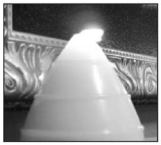mycollegehive
Approximate how much wax, to the nearest cubic centimeter, is needed to make this candle
JMAP geometry math cone0A candle maker uses a mold to make candles like the one shown below.The height of the candle is 13 cm and the circumference of the candle at its widest measure is 31.416 cm. Use modeling to approximate how much wax, to the nearest cubic centimeter, is needed to make this candle. Justify your answer.

32 viewsShareFollowUniversity of Lagos Nigeria
23 June 2020University of Benin Nigeria
23 June 20200The diagram above is a cone and the volume of a cone is given as: V = 1/3 πr2h.

Where r and h are the radius and height of the cone respectively.

Also, since the base of the cone is a circle, its circumference is given as: C = 2πr

Thus, 31.416 = 2πr

Divide both sides by 2π

r = 31.416/2π

r = 5

Therefore, V = 1/3 π(5)2(13)

V = 340 cubic centimeterShare### Related Tags

JMAP

2 followers

141 questionsgeometry

2 followers

99 questionsmath

2 followers

988 questions